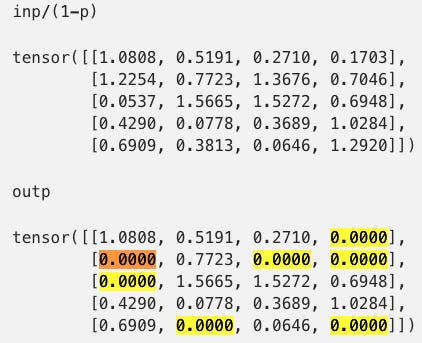# Scaling in Neural Network Dropout Layers (with Pytorch code example)

Source: Deep Learning on MediumScaling in dropout

For several times I confused myself over how and why a dropout layer scales its input. I’m writing down some notes before I forget again.

Link to Jupyter notebook:

In Pytorch doc it says:

Furthermore, the outputs are scaled by a factor of 1/(1-p) during training. This means that during evaluation the module simply computes an identity function.

So how is this done and why? Let’s look at some code in Pytorch.

Create a dropout layer `m` with a dropout rate `p=0.4`:

`import torch`
`import numpy as np`
`p = 0.4`
`m = torch.nn.Dropout(p)`

As explained in Pytorch doc:

During training, randomly zeroes some of the elements of the input tensor with probability `p` using samples from a Bernoulli distribution. The elements to zero are randomized on every forward call.

Put a random input through the dropout layer and confirm that ~40% (`p=0.4`) of the elements have become 0:

`nbig = 5000000`
`inp = torch.rand(nbig, 10)`
`outp = m(inp)`
`print(f'percent of zero elements in the output: {(outp==0).numpy().mean():.5f}, is close to p={p}')`
`percent of zero elements in the output: 0.40007, is close to p=0.4`

We now look at the scaling part:

Furthermore, the outputs are scaled by a factor of 1/(1-p) during training.

Create a smaller random input and put it through the dropout layer. Compare input and output:

`np.random.seed(42)`
`inp = torch.rand(5, 4)`
`inp`
`tensor([[0.6485, 0.3114, 0.1626, 0.1022],        [0.7352, 0.4634, 0.8206, 0.4228],        [0.0322, 0.9399, 0.9163, 0.4169],        [0.2574, 0.0467, 0.2213, 0.6171],        [0.4146, 0.2288, 0.0388, 0.7752]])`

We can see below that the outputs are scaled by a factor of 1/(1-p) during training, by comparing the non-zero elements in the two tensors below:

`outp = m(inp)`
`inp/(1-p)`
`tensor([[1.0808, 0.5191, 0.2710, 0.1703],        [1.2254, 0.7723, 1.3676, 0.7046],        [0.0537, 1.5665, 1.5272, 0.6948],        [0.4290, 0.0778, 0.3689, 1.0284],        [0.6909, 0.3813, 0.0646, 1.2920]])`
`outp`
`tensor([[1.0808, 0.5191, 0.2710, 0.0000],        [0.0000, 0.7723, 0.0000, 0.0000],        [0.0000, 1.5665, 1.5272, 0.6948],        [0.4290, 0.0778, 0.3689, 1.0284],        [0.6909, 0.0000, 0.0646, 0.0000]])`

We can assert that observation in code:

`idx_nonzero = outp!=0`
`assert np.allclose(outp[idx_nonzero].numpy(), (inp/(1-p))[idx_nonzero].numpy())`

So why does it does this? In the doc:

This means that during evaluation the module simply computes an identity function.

This basically says during evaluation/test/inference time, the dropout layer becomes an identity function and makes no change to its input.

Because dropout is active only during training time but not inference time, without the scaling, the expected output would be larger during inference time because the elements are no longer being randomly dropped (set to 0). But we want the expected output with and without going through the dropout layer to be the same. Therefore, during training, we compensate by making the output of the dropout layer larger by the scaling factor of `1/(1−p)`. A larger `p` means more aggressive dropout, which means the more compensation we need, i.e. the larger the scaling factor `1/(1−p)`.

​The code below demonstrates how scaling factor put output back to the same scale as the input.

`inp = torch.rand(nbig, 10)`
`outp = m(inp)`
`print(f'Average output ({outp.mean():.4f}) of the dropout layer is close to averge input ({inp.mean():.4f}).')`
`Average output (0.5000) of the dropout layer is close to averge input (0.5000).`

Instead of making the output of the dropout layer larger during training, one could equivalently make the output of the identity function during inference smaller. However the former is easier to implement. There is a discussion on StackOverflow that provides some details. But be careful, `p` in that discussion (from slides of Standford CS231n: Convolutional Neural Networks for Visual Recognition) is the ratio for keeping instead of for dropping.